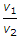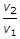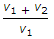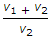# Mechanical Engineering - Thermodynamics

Exercise : Thermodynamics - Section 2
26.
Liquid fuels have lower efficiency than solid fuels.
True
False
Explanation:
No answer description is available. Let's discuss.

27.
The heat absorbed during a polytropic process is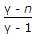x Workdone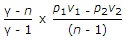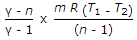all of these
Explanation:
No answer description is available. Let's discuss.

28.
Liquid fuels consist of hydrocarbons.
True
False
Explanation:
No answer description is available. Let's discuss.

29.
The expansion ratio (r) is the ratio of (where v1 = Volume at the beginning of expansion, and v2 = Volume at the end of expansion)